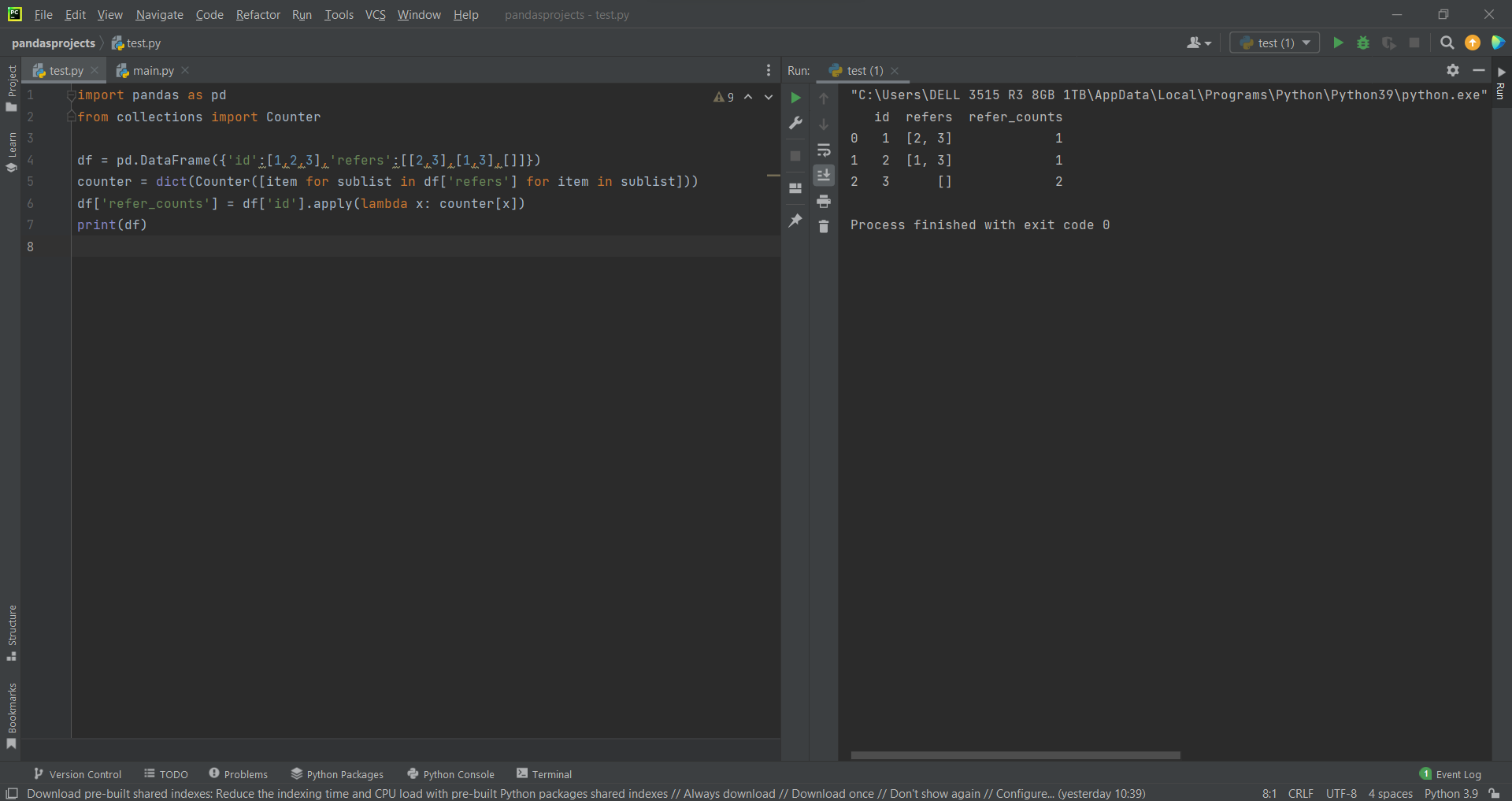# Array Operation of a Cell in Pandasby Abdul Rawoof A RUpdated: Feb 10, 2023Solution Kit

Array operations are mathematical and logical operations that can be performed on arrays, including numpy arrays and pandas DataFrames. These operations are used to manipulate and transform data and are an important part of data analysis and scientific computing.

In pandas, you can perform various array operations on a specific cell of a DataFrame by using the ".at[]" or ".iat[]" attributes. The basic syntax for both is similar, where the first argument is the index or label of the row, and the second argument is the label or index of the column.

• .at[]: This attribute allows you to access a single cell of a DataFrame by using the row and column labels.
• .iat[]: This attribute allows you to access a single cell of a DataFrame by using the integer index of the row and column.

Once you have accessed the cell, you can perform various array operations, such as mathematical, logical, or bitwise operations. For example, you can perform mathematical operations like +, -, *, /, ** and logical operations like ==, !=, <, >, <=, >=, &, |, ~ and bitwise operations like &, |, ^.Fig : Preview of the output that you will get on running this code from your IDE.

### Code

In this solution we're using Pandas library.

### Instructions

Follow the steps carefully to get the output easily.

2. Copy the snippet using the 'copy' and paste it in your IDE.
3. Add required dependencies and import them in Python file.
4. Add print statement at end of the code(like 'print(df)', also refer preview of the output).
5. Run the file to generate the output.

I hope you found this useful. I have added the link to dependent libraries, version information in the following sections.

I found this code snippet by searching for 'How to do array operation of a cell in Pandas' in kandi. You can try any such use case!

### Environment Tested

I tested this solution in the following versions. Be mindful of changes when working with other versions.

1. The solution is created in PyCharm 2021.3.
2. The solution is tested on Python 3.9.7.
3. Pandas version-v1.5.2.

Using this solution, we are able to do array operation of a cell in pandas with simple steps. This process also facilities an easy way to use, hassle-free method to create a hands-on working version of code which would help us to do array operation of a cell in pandas.

### Dependent Library

pandasby pandas-dev

Python37363Version:v2.0.0rc1Flexible and powerful data analysis / manipulation library for Python, providing labeled data structures similar to R data.frame objects, statistical functions, and much more

Support
Quality
Security
Reuse

pandasby pandas-dev

Python37363Version:v2.0.0rc1License: Permissive (BSD-3-Clause)

Flexible and powerful data analysis / manipulation library for Python, providing labeled data structures similar to R data.frame objects, statistical functions, and much more
Support
Quality
Security